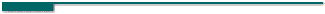Guide to Constraint Programming © Roman Barták, 1998 Contents Prev Up Next

# Over-Constrained Problems

In many real-life applications, there does not exist solution, i.e., valuation of variables that satisfies all the constraints. Such systems of constraints are called over-constrained systems, opposite to under-constrained systems of constraints that have many solutions satisfying all the constraints.

Example: Consider the problem of choosing matching clothes (shirt, shoes and trousers) that can be easily modeled using three finite domain variables with a number of binary constraints between them:
shirts S::{r,w} for red and white respectively,
shoes (footwear)
F::{c,s} for cordovans and sneakers
trousers
T::{b,d,g} for blue, denim, and grey

matching constraints (CSF denotes matching pairs of shirts and shoes):

CST::{(r,g),(w,b),(w,d)}
CFT::{(s,d),(c,g)}
CSF::{(w,c)}.

Visibly, this problem is over-constrained; it has no solutions. Therefore we need to consider some way of relaxing or weakening the problem until solutions can be found.

The traditional algorithms for constraint satisfaction are not able solve over-constrained systems although the stochastic algorithms can maximize the number of satisfied constraints. Therefore, some alternative approaches have been proposed to solve over-constrained systems or to generalize the notion of constraint respectively.###Extending CSP

The constraints in classical constraint satisfaction problems are crisp, i.e., they allow a tuple (of values of involved variables) or not. Alternative approaches to constraint satisfaction were proposed to enable non-crisp constraints, probabilities or weights respectively.

###Partial Constraint Satisfaction Problems

The Partial Constraint Satisfaction (PCSP) scheme of Freuder and Wallace is an interesting extension of CSP, which allows the relaxation and optimization of problems via weakening the original CSP.

###Constraint Hierarchies

Constraint hierarchies have been proposed to describe over-constrained systems of constraints by specifying constraints with hierarchical preferences, i.e., hard and soft constraints. While the hard (required) constraints must hold, the soft (preferential) constraints should be satisfied as much as possible depending on the criterion used.

###Algorithms for Solving Constraint Hierarchies

Overview of algorithms for solving constraint hierarchies (refining algorithm - DeltaStar; local propagation - DeltaBlue, SkyBlue, Indigo, Houria; others - IHCS, projection).

###Alternative and Generalized Approaches

To model features of various CSP systems, the general frameworks have been proposed. Among them the compositional theory for reasoning about over-constrained systems and the semiring-based constrained satisfaction are the most popular.

 Contents Prev Up Next Designed and maintained by Roman Barták